# 05 Question (3 points) See page 678 Acetic acid (CH3COOH) and trifluoroacetic acid (CF3COOH) are shown...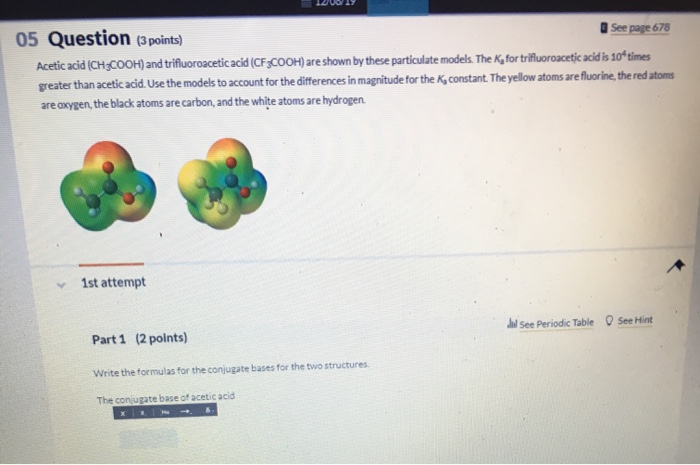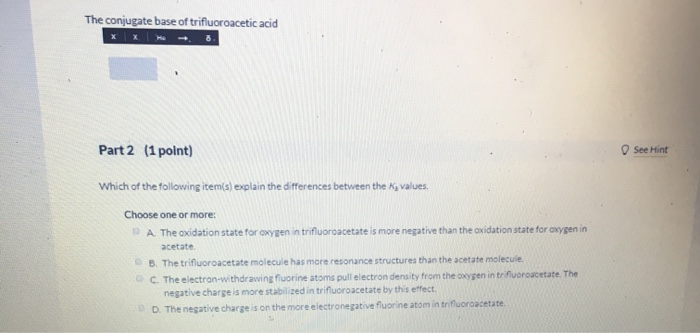05 Question (3 points) See page 678 Acetic acid (CH3COOH) and trifluoroacetic acid (CF3COOH) are shown by these particulate models. The K, for trifluoroacetic acid is 10ʻtimes greater than acetic acid. Use the models to account for the differences in magnitude for the K, constant. The yellow atoms are fluorine, the red atoms are oxygen, the black atoms are carbon, and the white atoms are hydrogen 1st attempt See Periodic Table O See Hint Part 1 (2 points) Write the formulas for the conjugate bases for the two structures The coniugate base of acetic acid
The conjugate base of trifluoroacetic acid Part 2 (1 point) See Hint Which of the following item(s) explain the differences between the Ky values Choose one or more: A The oxidation state for oxygen in trifluoroacetate is more negative than the oxidation state for oxygen in acetate B. The trifluoroacetate molecule has more resonance structures than the acetate molecule C. The electron-withdrawing fluorine atoms pull electron density from the oxygen in trifluoroacetate. The negative charge is more stabilized in trifluoroacetate by this effect. D. The negative charge is on the more electronegative fluorine atom in trifluoroacetate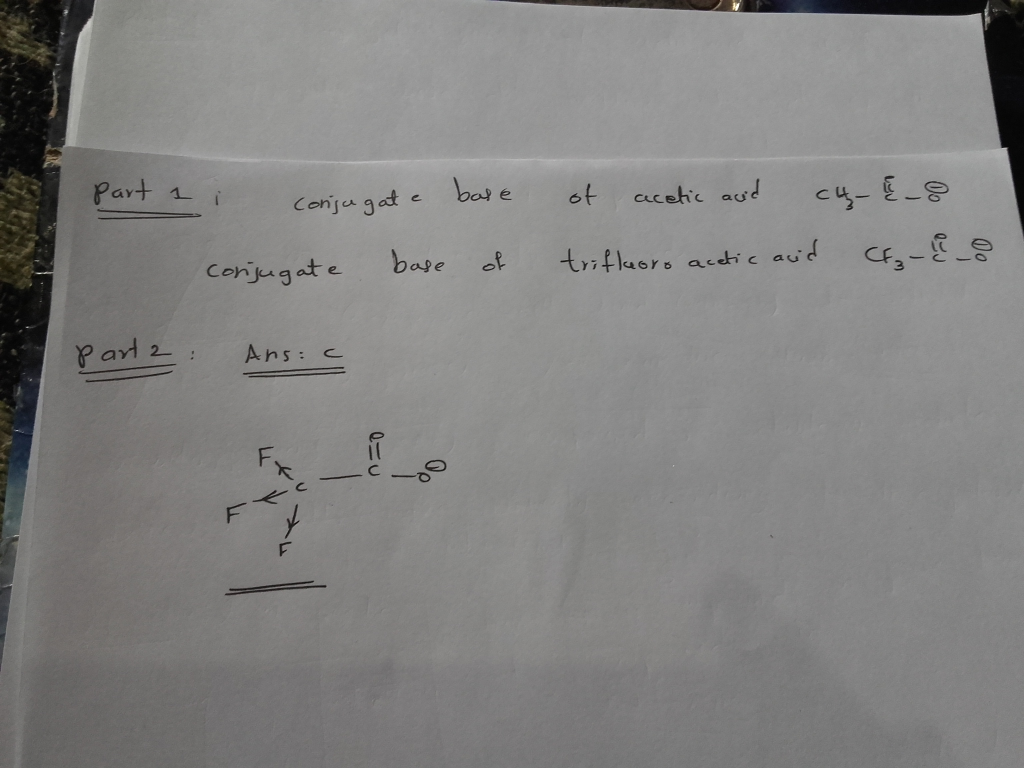#### Earn Coin

Coins can be redeemed for fabulous gifts.

Similar Homework Help Questions
• ### 13 Question (3 points) Acetic acid (CH3COOH) and trifluoroacetic acid (CF3COOH) are shown by these particulate...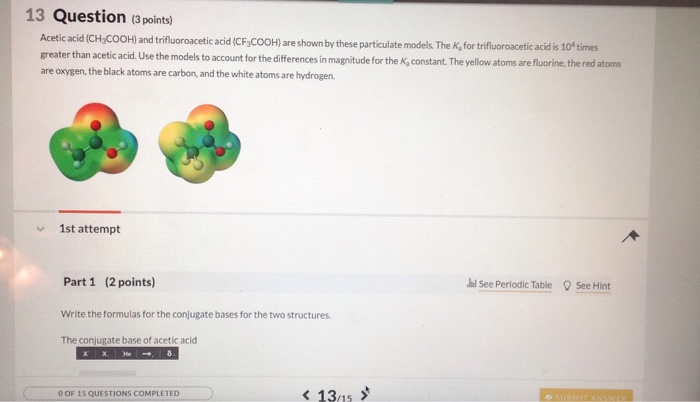13 Question (3 points) Acetic acid (CH3COOH) and trifluoroacetic acid (CF3COOH) are shown by these particulate models. The K, for trifluoroacetic acid is 10 times greater than acetic acid. Use the models to account for the differences in magnitude for the K, constant. The yellow atoms are fluorine, the red atoms are oxygen, the black atoms are carbon, and the white atoms are hydrogen 1st attempt Part 1 (2 points) See Periodic Table See Hint Write the formulas for the...

• ### Acetic acid (CH:COOH) and trifluoroacetic acid (CF COOH) are shown by these particulate models. The K,...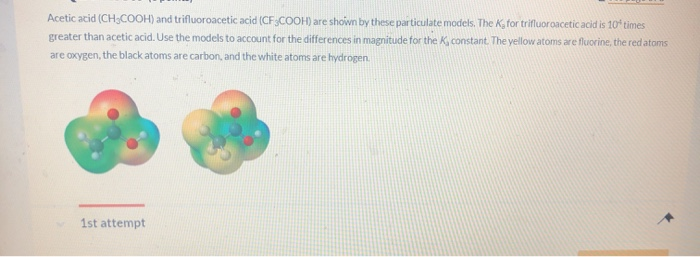Acetic acid (CH:COOH) and trifluoroacetic acid (CF COOH) are shown by these particulate models. The K, for trifluoroacetic acid is 10 times greater than acetic acid. Use the models to account for the differences in magnitude for the K, constant. The yellow atoms are fluorine, the red atoms are oxygen, the black atoms are carbon, and the white atoms are hydrogen. 1st attempt Part 1 (2 points) Write the formulas for the conjugate bases for the two structures. The conjugate...

• ### 07 Question (1 point) a See page 729 Acetic acid and its conjugate base acetate can...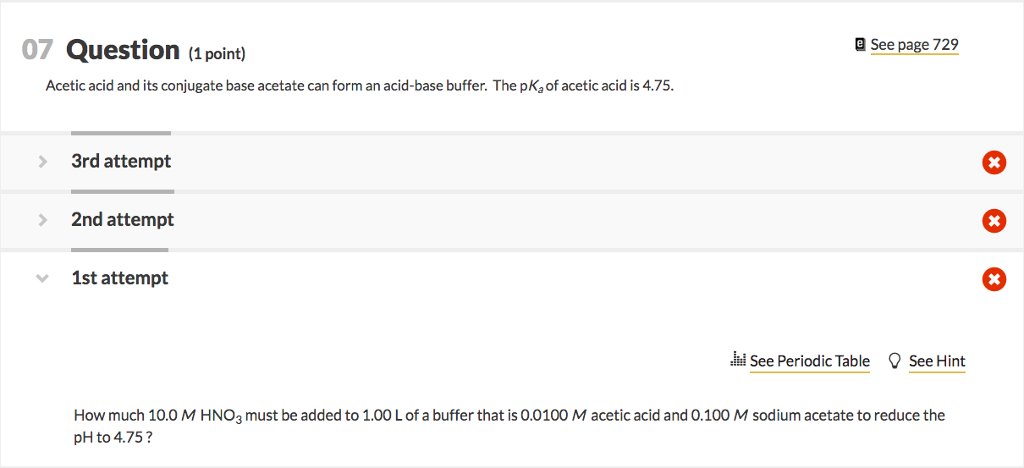07 Question (1 point) a See page 729 Acetic acid and its conjugate base acetate can form an acid-base buffer. The pKa of acetic acid is 4.75. 3rd attempt 2nd attempt 1st attempt ded See Periodic Table See Hint How much 10.0 M HNO3 must be added to 1.00 L of a buffer that is 0.0100 M acetic acid and 0.100 M sodium acetate to reduce the pH to 4.75?

• ### Please answer all 3 questions QUESTION 29 Chloroacetic acid is stronger than acetic acid. Which is...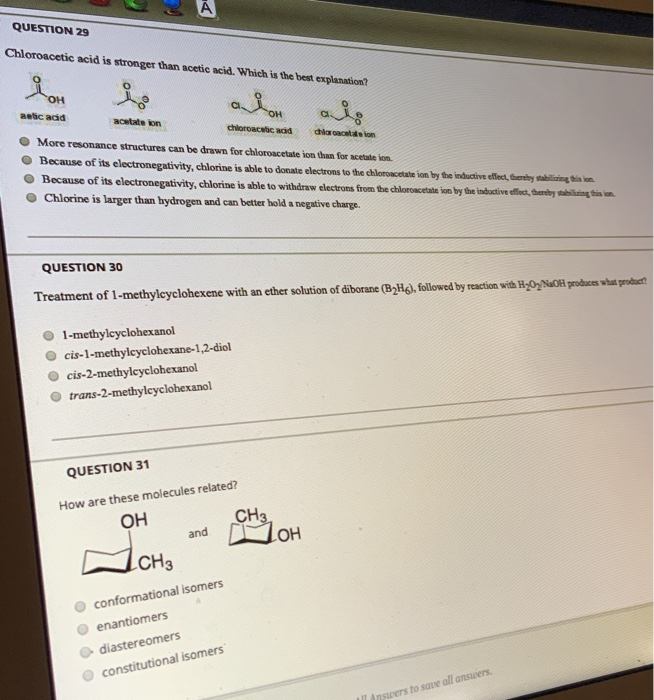Please answer all 3 questions QUESTION 29 Chloroacetic acid is stronger than acetic acid. Which is the best explanation? وله OH OH aetic add acetate kon chloroacetic acid location More resonance structures can be drawn for chloroacetate ion than for acetate in Because of its electronegativity, chlorine is able to donate electrons to the chloroacetate ion by the inductive effect, thereby stabilizing tim Because of its electronegativity, chlorine is able to withdraw electrons from the chloroacetate ion by the inductive...

• ### QUESTION 3 Salicylic acid reacts with acetic anhydride to form aspirin and acetic acid as shown. Use the equation to answer Questions 3 – 6.       (salicylic acid)                                   ...

QUESTION 3 Salicylic acid reacts with acetic anhydride to form aspirin and acetic acid as shown. Use the equation to answer Questions 3 – 6.       (salicylic acid)                                               (aspirin)                C7H6O3 (s) + C4H6O3 (l) → C9H8O4 (s) + CH3COOH (l)   MM = 138 MM = 180   If we divide 180 by 138 we find that 1.00 g salicylic acid will produce a maximum of 1.30 g aspirin. 3. A student starts with 3.20 g salicylic acid. What is the maximum amount...

• ### Titration: Acids and Bases 2. How can you determine which acid is diprotic? 3. using the...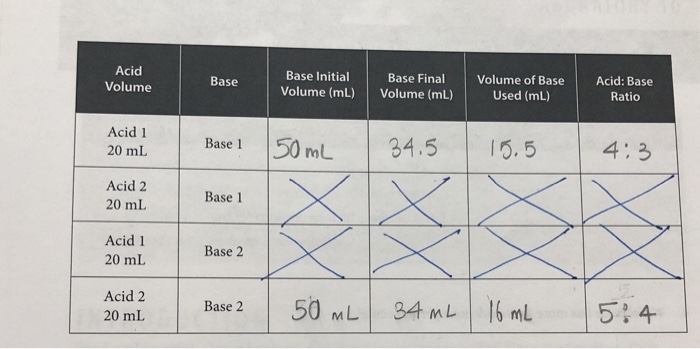Titration: Acids and Bases 2. How can you determine which acid is diprotic? 3. using the answers to questions one and two, which acid is diprotic? 4. Which base has more hydroxide ions per molecule? Acid Volume Base Base Initial Volume (mL) Base Final Volume (mL) Volume of Base Used (mL) Acid: Base Ratio Acid 1 20 mL Base 1 50 mL 34.5 15.5 4:3 Acid 2 20 mL Base 1 Acid 1 20 mL Base 2 Acid 2 20...

• ### e. 18 Test Your Knowledge MULTIPLE CHOICE: Choose the one best answer. 1. Each element has...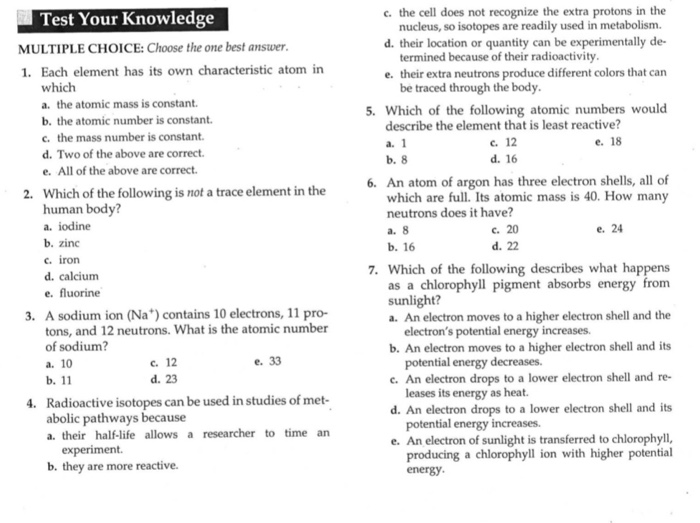e. 18 Test Your Knowledge MULTIPLE CHOICE: Choose the one best answer. 1. Each element has its own characteristic atom in which a. the atomic mass is constant. b. the atomic number is constant. c. the mass number is constant. d. Two of the above are correct. e. All of the above are correct. 2. Which of the following is not a trace element in the human body? a. iodine b. zinc c. iron d. calcium e. fluorine 3. A...

• ### I have a chemistry test that I need help with

I have a chemistry test that I need help with. My answers have stars by them Can someone please help me with the answers to this test??? Part 1 – Matter as Energy & Structure 1. When a pair of electrons is shared between two atoms, a covalent bond is said to have formed. Most often, the pair is formed a. by excess valence electrons b. as one atom contributes both electrons* c. as each atom contributes one electron d....

• ### answers them all . Bromination .table and 3-4 question too. BS132 Experiment (b) Bromination of Alkenes...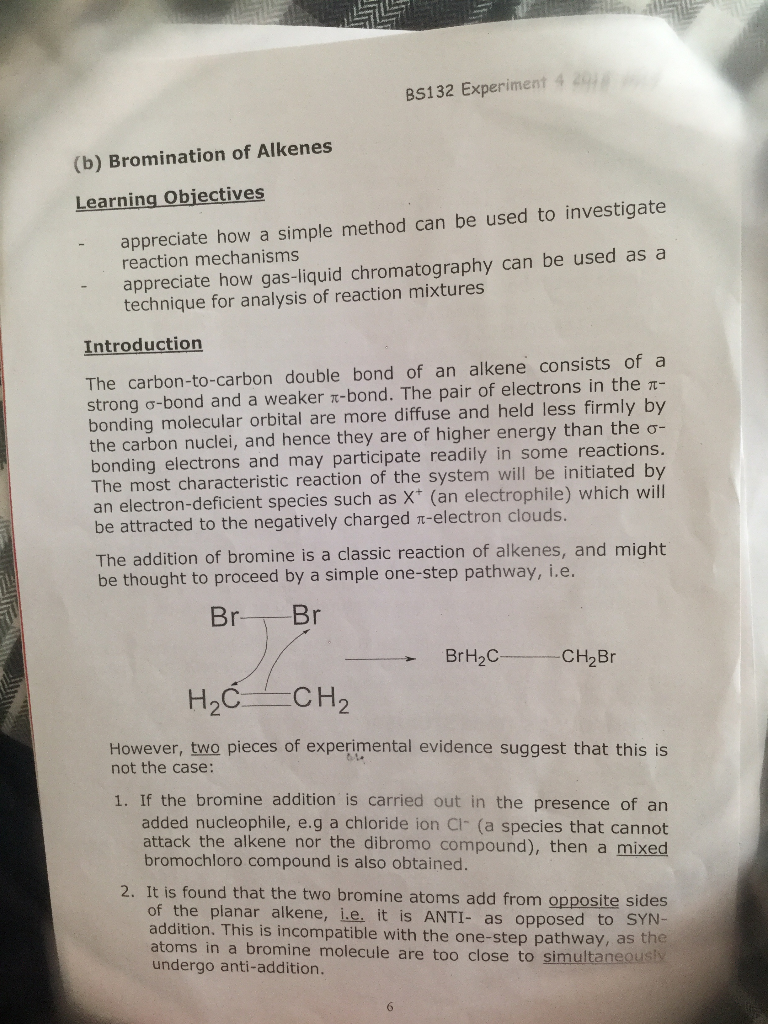answers them all . Bromination .table and 3-4 question too. BS132 Experiment (b) Bromination of Alkenes Learning Objectives appreciate how a simple method can be used to investigate reaction mechanisms appreciate how gas-liquid chromatography can be used asa technique for analysis of reaction mixtures Introduction The carbon-to-carbon double bond of an alkene consists ofa strong σ-bond and a weaker π-bond. The pair of electrons in the t- bonding molecular orbital are more diffuse and held less firmly by the carbon...

Free Homework App